# Hypercubes and Graphs

The adjacency matrix of a hypercube demonstrates the new MATLAB graph object.

### Contents

#### Graphs

My previous blog post was about the Patience Chinese Rings Puzzle. The puzzle's state lives in a six-dimensional space. There are 64 vertices, numbered 0 from to 63. Each vertex is connected to six other vertices, the ones whose numbers, expressed in binary, differ from its own by one bit. That connectivity arrangement -- those vertices and the connections between them -- form a graph known as a hypercube.

Recent versions of MATLAB include two new objects, graph and digraph. Here is an abbreviated help entry for graph.

>> help graph
graph Undirected Graph
G = graph(A) uses the square symmetric matrix A as an adjacency matrix
and constructs a weighted graph with edges corresponding to the nonzero
entries of A.  If A is logical then no weights are added.
G = graph(A,NAMES) additionally uses the cell array of strings NAMES as
the names of the nodes in G.  NAMES must have as many elements as
size(A,1).

#### Hypercubes

And here is a function that generates the adjacency matrix for a hypercube.

   type hypercube

function A = hypercube(d)
% hypercube(d) is a logical adjacency matrix for a d-dimensional hypercube.
pow2 = 2.^(0:d-1);
f2b = @(j) floor(rem(j./pow2,2)); % flint to binary
b2f = @(b) b*pow2'; % binary to flint
n = 2^d;
A = zeros(n,n,'logical');
for j = 0:n-1
% Convert column index to binary.
b = f2b(j);
% Flip bits to get corresponding row indices.
for i = 1:d
b(i) = ~b(i);
k = b2f(b);
b(i) = ~b(i);
A(k+1,j+1) = 1;
end
end
end


#### IPSC

I first encountered hypercubes thirty years ago. For a couple of years in the late 1980s I was involved with one of the world's first commercial parallel computers, the IPSC, the Intel Personal Super Computer. Here is a link to my blog post in 2013.

The IPSC was constructed from single board computers, similar to the mother boards in the PCs of the day. We had three models -- d5, d6 and d7 -- with 32, 64 or 128 processors. Since it was impractical to connect every processor directly to every other processor, the connection network formed a hypercube. For a machine with $n = 2^d$ processors, that's $n \log_2{n}$ instead of $n^2$ connections. For the d6 that's 384 connections instead of 4096.

The d6 model has the same graph as the patience puzzle that I wrote about in my previous post. (Actually, the IPSC's graph is a directed graph, a digraph, because the connections are two-way. But undirected graphs have simpler plots.)

#### 3d

A 3-dimensional hypercube is not a conventional cube. The 3-d hypercube is only the vertices and edges of the cube. The cube itself includes its surfaces and interior. If you had a wire-frame cube made from rubber bands and squished it around without destroying the connectivity, you wouldn't have a cube any more, but you would still have a model of a hypercube.

Here is the adjacency matrix of a 3-d hypercube. (It's so small that it's not necessary to make use of the sparsity.)

   A = hypercube(3)

A =
8×8 logical array
0   1   1   0   1   0   0   0
1   0   0   1   0   1   0   0
1   0   0   1   0   0   1   0
0   1   1   0   0   0   0   1
1   0   0   0   0   1   1   0
0   1   0   0   1   0   0   1
0   0   1   0   1   0   0   1
0   0   0   1   0   1   1   0


One view of this matrix is its spy plot.

   spy(A)To get other views, let's use the new MATLAB graph object. We'll label the nodes with '0' through '7'.

   nodes = num2cellstr(0:7)
G = graph(A,nodes)

nodes =
1×8 cell array
'0'    '1'    '2'    '3'    '4'    '5'    '6'    '7'
G =
graph with properties:

Edges: [12×1 table]
Nodes: [8×1 table]


#### Layouts

By itself, a graph doesn't have any geometric structure. In order to plot it you specify a layout, or coordinates, for the nodes. Coming up with good layouts is an art, as well as a science. Here are the currently available layouts.

     'auto'      (Default) Automatic choice of layout method based on
the structure of the graph.
'circle'    Circular layout.
'force'     Force-directed layout. Uses attractive and repulsive
forces on nodes.
'layered'   Layered layout. Places nodes in a set of layers.
'subspace'  Subspace embedding layout. Uses projection onto an
embedded subspace.
'force3'    3-D force-directed layout.
'subspace3' 3-D Subspace embedding layout.

The default layout of the 3-d hypercube graph is a perspective view of an ordinary cube.

   plot(G)
axis square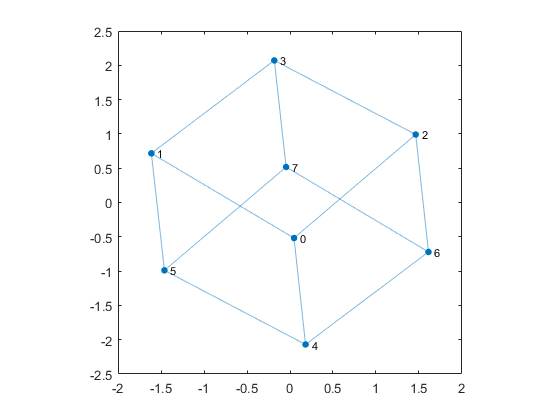#### 4d

Let's move up to four dimensions.

   A = hypercube(4);
spy(A)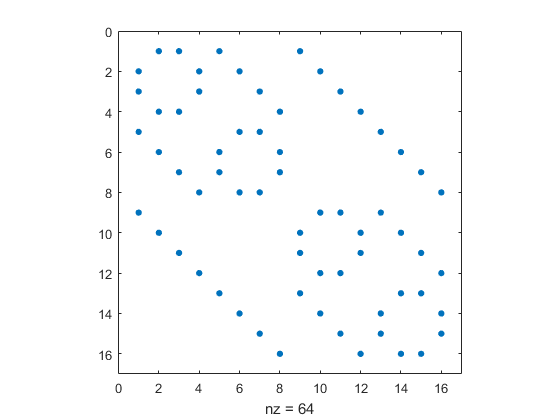I think the default layout of the 4-d hypercube is attractive.

   nodes = num2cellstr(0:15)';
G = graph(A,nodes);
plot(G)
axis square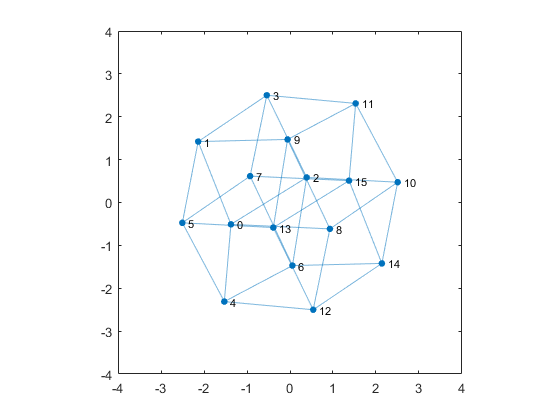But 'circle' is another attractive layout. Notice that '3' is not connected to '4' because 0011 and 0100 differ by more than a single bit. The blue dot in the center is not a node -- it is just the point where many edges cross.

   plot(G,'layout','circle')
axis square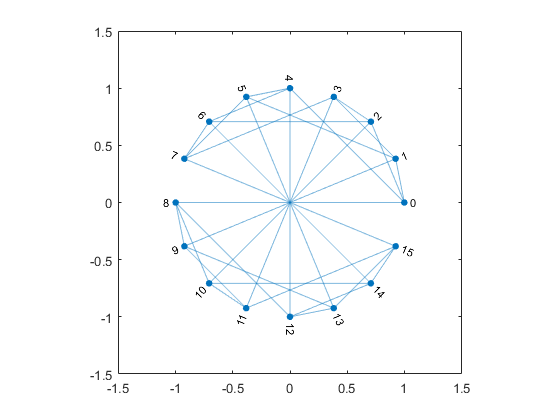Here is yet another view.

   plot(G,'layout','layered')
axis square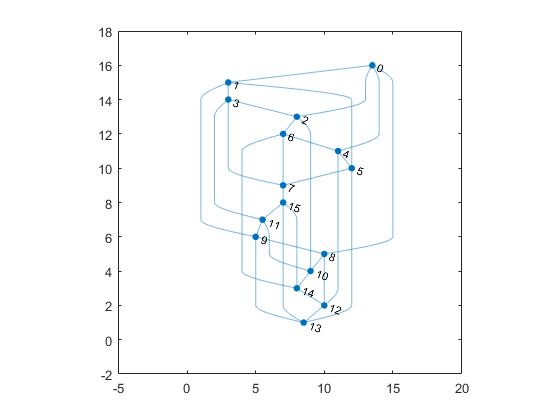#### Insider

I'm still not satisfied. I want one cube inside of another. I can supply my own coordinates for the vertices.

    type cubelet

function x = cubelet
% x = cubelet
% 8-by-3 array of vertices of a unit cube.
x = [-1 -1 -1
1 -1 -1
-1  1 -1
1  1 -1
-1 -1  1
1 -1  1
-1  1  1
1  1  1];
end

    x = cubelet;
x = [3*x; x];
plot(G,'xdata',x(:,1),'ydata',x(:,2),'zdata',x(:,3))
axis equal off
view(-20,11)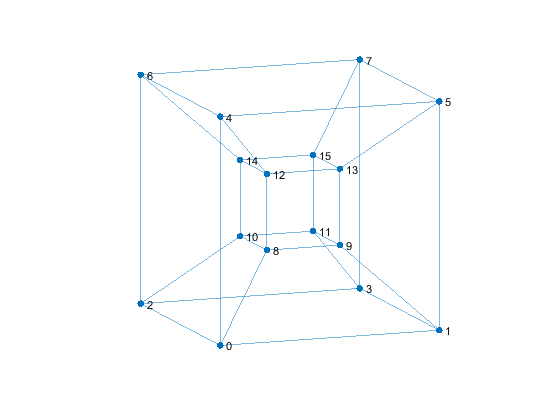This served as a logo for the IPSC.

#### Eigenvectors

The Laplacian of a graph is a matrix that has the degree of the nodes on the diagonal and -1's off the diagonal marking the edges. For the 3-d hypercube the degrees are all 4. So here the Laplacian.

    L = full(laplacian(G));
frmt = ['   ' repmat('%3d',1,16) '\n'];
fprintf('L = \n')
fprintf(frmt,L)

L =
4 -1 -1  0 -1  0  0  0 -1  0  0  0  0  0  0  0
-1  4  0 -1  0 -1  0  0  0 -1  0  0  0  0  0  0
-1  0  4 -1  0  0 -1  0  0  0 -1  0  0  0  0  0
0 -1 -1  4  0  0  0 -1  0  0  0 -1  0  0  0  0
-1  0  0  0  4 -1 -1  0  0  0  0  0 -1  0  0  0
0 -1  0  0 -1  4  0 -1  0  0  0  0  0 -1  0  0
0  0 -1  0 -1  0  4 -1  0  0  0  0  0  0 -1  0
0  0  0 -1  0 -1 -1  4  0  0  0  0  0  0  0 -1
-1  0  0  0  0  0  0  0  4 -1 -1  0 -1  0  0  0
0 -1  0  0  0  0  0  0 -1  4  0 -1  0 -1  0  0
0  0 -1  0  0  0  0  0 -1  0  4 -1  0  0 -1  0
0  0  0 -1  0  0  0  0  0 -1 -1  4  0  0  0 -1
0  0  0  0 -1  0  0  0 -1  0  0  0  4 -1 -1  0
0  0  0  0  0 -1  0  0  0 -1  0  0 -1  4  0 -1
0  0  0  0  0  0 -1  0  0  0 -1  0 -1  0  4 -1
0  0  0  0  0  0  0 -1  0  0  0 -1  0 -1 -1  4


The Laplacian could also be obtained from the original adjacency matrix.

    L = diag(sum(A)) - A;


The coordinates of possible layouts for the plot of the graph can be obtained by picking three of the eigenvectors of the Laplacian. Here are all of the eigenvalues and three of the eigenvectors. (The eigenvalues are all integers.)

    [V,E] = eig(L);
evals = round(diag(E)');
fprintf('evals = \n')
fprintf(frmt,evals)
evecs3 = V(:,1:3)

evals =
0  2  2  2  2  4  4  4  4  4  4  6  6  6  6  8
evecs3 =
-0.2500    0.0216    0.0025
-0.2500    0.1620    0.4067
-0.2500    0.0245   -0.1449
-0.2500    0.1649    0.2593
-0.2500    0.2546   -0.2521
-0.2500    0.3950    0.1521
-0.2500    0.2575   -0.3996
-0.2500    0.3979    0.0046
-0.2500   -0.3979   -0.0046
-0.2500   -0.2575    0.3996
-0.2500   -0.3950   -0.1521
-0.2500   -0.2546    0.2521
-0.2500   -0.1649   -0.2593
-0.2500   -0.0245    0.1449
-0.2500   -0.1620   -0.4067
-0.2500   -0.0216   -0.0025


And here is the resulting plot.

    G = graph(A,nodes);
plot(G,'xdata',V(:,1),'ydata',V(:,2),'zdata',V(:,3))
axis square off
set(gca,'clipping','off')
zoom(2)
view(-75,45)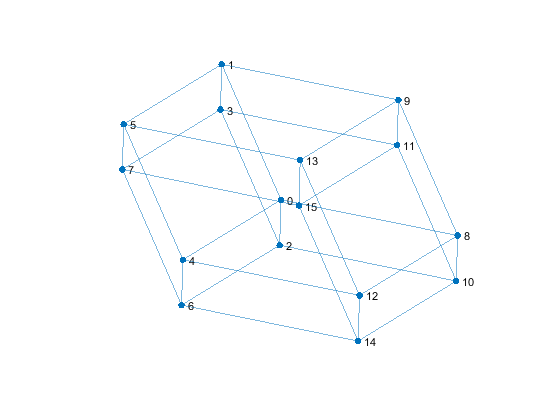#### 6d

The state space of both the puzzle that got me started on this blog post and one of the models of the parallel computer that consumed me thirty years ago is six dimensional.

    A = hypercube(6);
spy(A)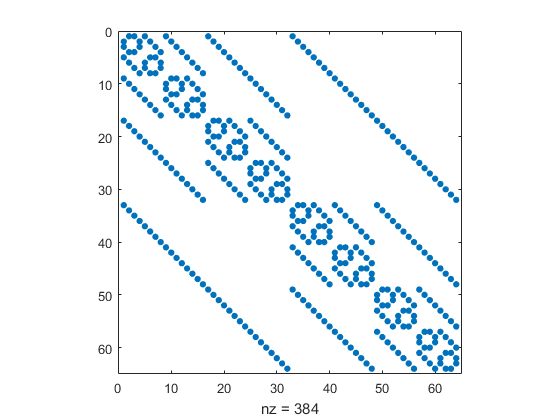A plot of a cube inside of a cube inside of a cube inside of a cube is a bit messy. The circle layout is an interesting design, but it is hard to see which nodes are connected to which.

    nodes = num2cellstr(0:63)';
G = graph(A,nodes);
plot(G,'layout','circle')
axis square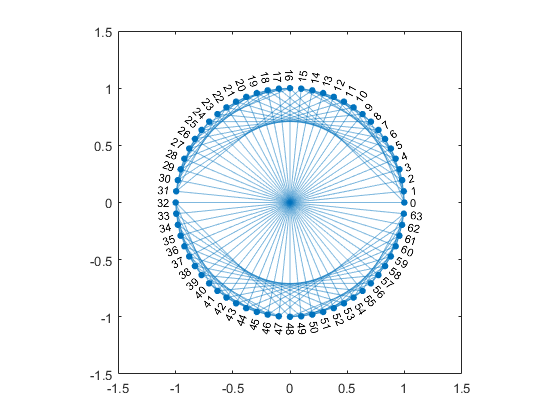Here is a three-dimensional layout.

    plot(G,'layout','subspace3')
axis off square
set(gca,'clipping','off')
zoom(2)
view(-108,-21)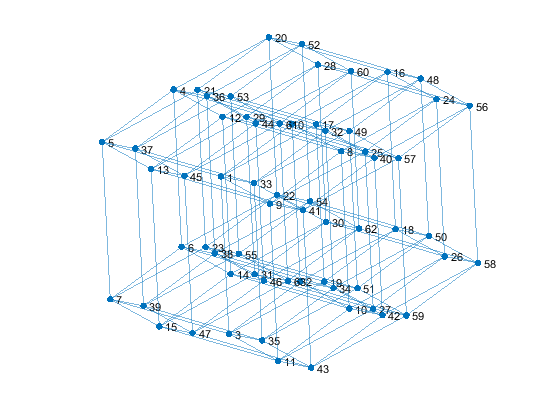#### Bend It Like Beckham

The Bucky Ball provides an elegant example of a graph. Here's the help entry.

   help bucky

 BUCKY  Connectivity graph of the Buckminster Fuller geodesic dome.
B = BUCKY is the 60-by-60 sparse adjacency matrix of the
connectivity graph of the geodesic dome, the soccer ball,
and the carbon-60 molecule.
[B,V] = BUCKY also returns xyz coordinates of the vertices.



When we put bucky into MATLAB years ago we went to a whole lot of trouble to compute those vertex coordinates. (To see how much trouble, type bucky.) But now, with the three dimensional force3 layout, the graph/plot function does that work. Putting a little spin on it makes it interesting to watch.

   type bucky_gif

B = graph(bucky);
plot(B,'layout','force3')
axis square off vis3d
set(gca,'clipping','off')
zoom(2)
[a,e] = view;
gif_frame('bucky_spin.gif')
for d = 10:10:360
view(a+d,e+d)
gif_frame
end
gif_frame('reset')It's more fun to use the cameratoolbar and put the spin on the ball yourself.

Published with MATLAB® R2017a

|